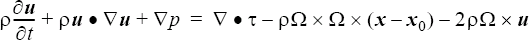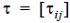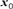# ROTATION_FORCE

Specifies rotational force resulting from use of rotating frame of reference (obsolete command).

AcuSolve Command

## Syntax

ROTATION_FORCE("name") {parameters...}

User-given name.

## Parameters

centrifugal (boolean) [=off]
Flag specifying whether to apply centrifugal force.
coriolis (boolean) [=off]
Flag specifying whether to apply coriolis force.
rotation_center or center (array) [={0,0,0}]
Center of rotation, specified in global xyz coordinate system. Used with centrifugal force.
angular_velocity or ang_vel (array) [={0,0,0}]
Vector of angular velocity of the rotating frame, in radians per unit of time.

## Description

This command is obsolete and will be removed eventually. The REFERENCE_FRAME command should be used instead. Note that using both commands at the same time will normally produce incorrect results.

This command specifies the body forces resulting from solving a problem in a rotating frame of reference. Only a constant rotation_center and a constant angular_velocity are supported. The centrifugal and coriolis terms appear as the last two terms in the momentum equation:(1)where $\rho$ is the density; u is the velocity vector; p is the pressure;is the viscous stress tensor; x is the current coordinate vector;is the center of rotation, given by rotation_center; and Ω is the angular rotation rate vector, given by angular_velocity. ROTATION_FORCE commands are referenced by BODY_FORCE commands, which in turn are referenced by ELEMENT_SET commands:
ROTATION_FORCE ( "rotating frame" ) {
centrifugal                         = on
coriolis                            = on
center                              = { 0, 0, 0 }
angular_velocity                    = { 0, 1, 0 }
}
BODY_FORCE( "my body force" ) {
rotation_force                      = "rotating frame"
...
}
ELEMENT_SET( "fluid in rotating frame of reference" ) {
body_force                          = "my body force"
...
}

In this example, the frame of reference rotates around the y axis at the rate of one radian per unit of time, with the axis of rotation centered at the point (0,0,0) in the global xyz coordinate system. The right hand rule is used to define the direction of rotation of the reference frame.